# MODE Excel FunctionArticle byExcelMojo Team## What Is MODE Function In Excel?

The MODE function in Excel determines the most frequently occurring value in a given data set. And if the supplied array contains two or more modes, the function returns the least mode value.

The MODE Excel function is an inbuilt Statistical function that users can use to find the mode of a given data range while performing financial evaluations. However, the functions MODE.MULT and MODE.SNGL replaced the MODE() in Excel 2010, offering improved accuracy. And the MODE() is now available as a Compatibility function in Excel for backward compatibility.

For example, the below table shows a list of smartphone models and their popularity in 2022 as percentage values in columns A and B, respectively.

Suppose we require to determine the most repetitive percentage value in column B and display the result in the target cell B9. Then, considering the MODE Excel function definition, we can apply the function in cell B9.

In the above MODE function example, the MODE() takes the data range B2:B6 as the input and determines the percentage value that occurs maximum times. And as the value 51% occurs twice in the list, while the remaining values occur only once, the function returns 51% as the mode value for the data range B2:B6.

Please note that the data format in cell B9 is Percentage, as highlighted in the above image.

###### Key Takeaways
• The MODE Excel function returns the most frequently appearing data point in the given data set as the mode value. Users can apply the MODE() to determine the mode of the data set required in financial calculations.
• The MODE() takes one mandatory number argument as input and can accept 2 to 254 optional number arguments.
• The MODE() gives excellent results when used with other Excel functions, such as INDEX and MATCH.
• The latest versions of the MODE(), MODE.SNGL() and MODE.MULT() are available in Excel in the Statistical functions category in the Formulas tab. They provide improved accuracy than the Compatibility function MODE.

### MODE() Excel Formula

The syntax of MODE Excel formula is:

where,

• number1: The first number we require to calculate the mode value for.
• number2,: The subsequent 2 to 254 number arguments for which we require to calculate the mode value.

While the first argument in the above MODE Excel formula is mandatory, the remaining number arguments are optional. And we can supply them as arguments (Excel cell references or array references) separated by commas or an array reference.

Please ensure we adhere to the following points when applying the MODE().

• The MODE Excel formula arguments can be numeric values, named ranges in Excel , arrays, or cell references to numeric values.
• If a provided argument is a text, logical value, or empty cell, the MODE() ignores it. However, it counts cells containing zero.
• Suppose the MODE Excel formula arguments are error values or texts that do not translate into numeric values. Then they can create errors in the mode value calculation.
• If the supplied data set contains no repetitive data points, the MODE Excel function return value will be the #N/A error.

### How To Use MODE Excel Function?

The steps to use the MODE Excel function are as follows:

1. First, ensure the source data is accurate and free from error values or texts that do not translate into numbers.
2. Then, select the target cell and enter the MODE().
3. Finally, press Enter to view the MODE return value.

Below is an example to clearly understand the above steps.

Consider the following table showing six test results over five days.

And suppose we require to determine the mode value for each test and display the results in column G.

Then, we can apply the MODE Excel function in the target cells G2:G7 and achieve the required mode values.

1. Select the target cell G2, enter the following MODE(), and press Enter.

=MODE(B2,C2,D2,E2,F2)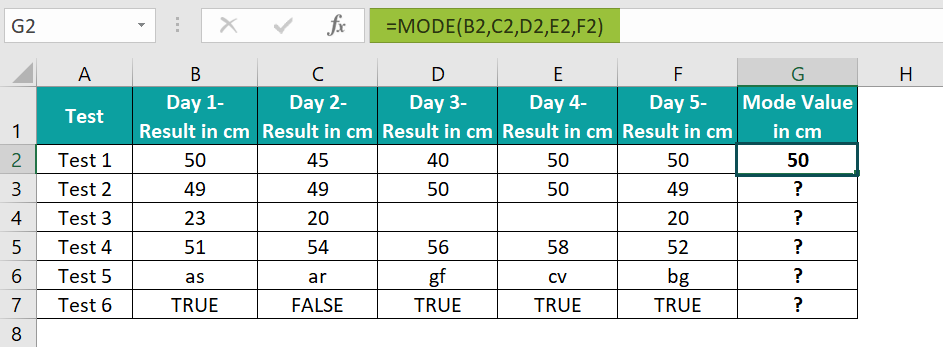Alternatively, we can select the target cell G2 and click FormulasMore FunctionsCompatibilityMODE to apply the MODE() through the Function Arguments window.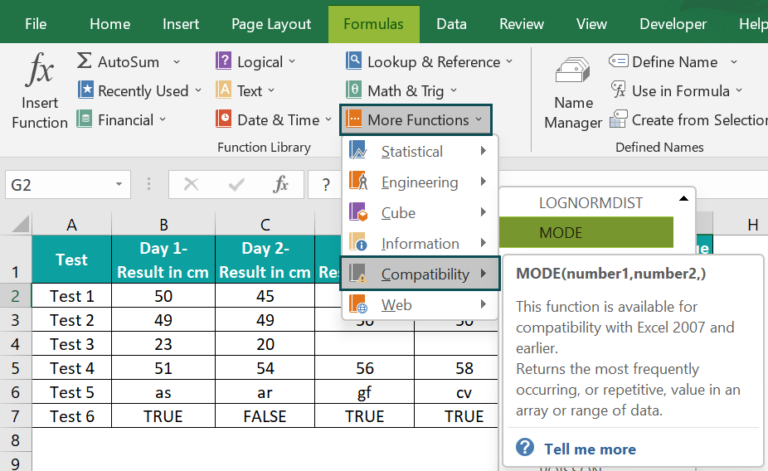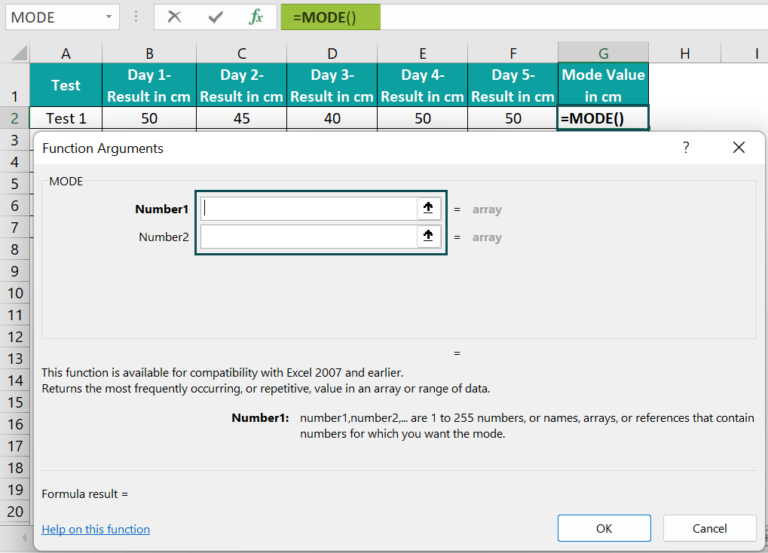Now, we can start entering the required numbers or cell references to the specific numbers as the arguments in the Function Arguments window. First, we will see the provision to enter two number arguments. But our data set contains five data points. So, to enter the remaining three values, click the second argument field. The window will show the field to enter the third argument. Likewise, we must click the successive number argument fields to supply the rest of the data points.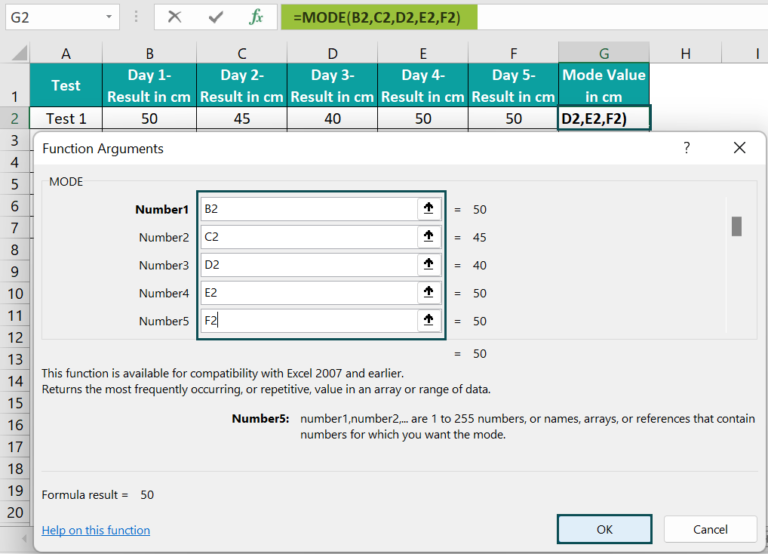And once we supply the required arguments and click OK, the MODE() gets executed. We can view the output, 50, in the target cell G2.

We can also enter the MODE() arguments as an array range, as shown below.

2. Select the target cell G3, enter the following MODE(), and press Enter.

=MODE(B3:F3)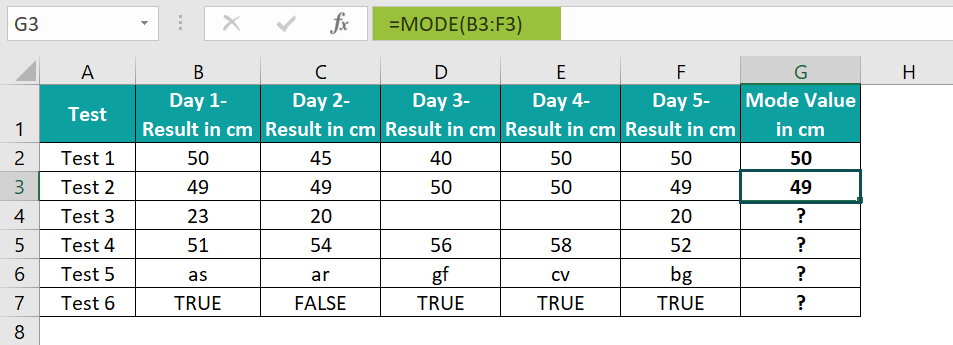Please Note: Suppose we must determine the mode for data values across multiple array ranges. Then, we can enter the array ranges separated by commas or the entire cell range that includes all the specific array ranges.
Now, we shall see what happens when we use the MODE() for data sets containing empty cells, texts, logical values, or no reoccurring data points.

3. Drag the fill handle downwards to copy the formula in cell range G4:G7.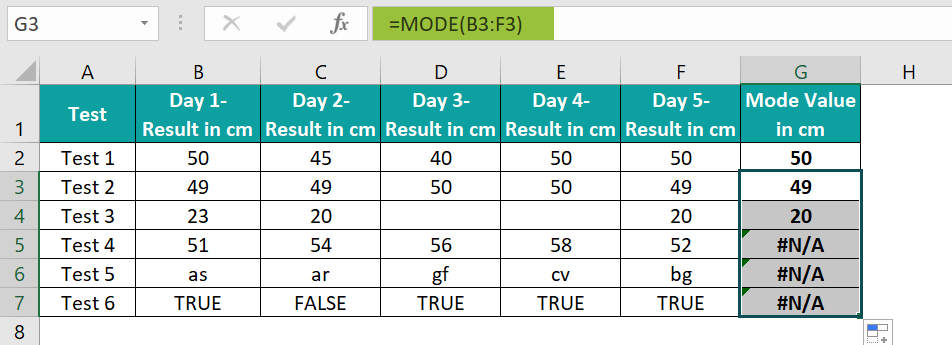In the above MODE Excel function example, row 4 contains empty cells. So, the function ignores them to return the number that appears most frequently, 20, as the output.

On the other hand, the above results show the #N/A error in cell range G5:G7. The reasons are:

• The data set in row 5 does not contain any reoccurring data points; hence, it has no mode value.
• The data set in row 6 contains texts that do not translate into numbers.
• The data set in row 7 contains only logical values, which the MODE() ignores, leading to the data set not containing any numbers for which the MODE() can find the mode value.

### Examples

Below are a few more examples to effectively explain the MODE Excel function definition.

#### Example #1

In this example, we will learn how the MODE Excel function behaves when a data set has more than one mode.

Assume the table below contains a list of tasks and the estimated days to complete each task.

We have to calculate the mode of the day values, provided in column B, to display it in the target cell E1. Then, here is how we can apply the MODE Excel function in cell E1 to get the required mode value.

Step 1: Select the target cell E1, enter the following MODE(), and press Enter.

=MODE(B2:B7)

The values 3 and 4 in the above data set in column B repeat twice. And thus, the data set has two mode values, 3 and 4. However, the MODE() returns the lowest mode; hence, the MODE() return value is 3 in this case.

#### Example #2

We can apply the MODE Excel function with other inbuilt Excel functions to achieve fruitful results.

For example, we can use the MODE() with INDEX and MATCH functions to determine the most repeatedly occurring word in a given list of words.

Consider the following table. It contains the list of the top 15 richest towns in the US and their corresponding states mentioned in columns A and B, respectively.

And suppose the requirement is to find the US state with the highest number of richest towns, based on the above list, and show the result in the target cell B19. Then here is how we can proceed to get the required state name.

Step 1: Select the target cell B19, enter the below formula containing the MODE Excel function, along with the INDEX and MATCH functions, and press Enter.

=INDEX(B2:B16,MODE(MATCH(B2:B16,B2:B16,0)))

In the above formula, the MATCH Excel function returns the relative position of each state in the array of state names, matching the specific state name in a specified order. So, it returns {1;2;1;4;1;6;7;8;9;7;6;2;13;9;15}.

Now, this array range becomes the input for the MODE(). And thus, it returns the value 1, as one occurs thrice in the array range.

Finally, the INDEX Excel function returns the value at the intersection of a specific row and column in the provided range. I

n this example, the range is B2:B16, and the row number is 1. And so, it returns the output California.

#### Example #3

Let us now see how the latest versions of the MODE Excel function, MODE.SNGL and MODE.MULT work.

The syntax for the functions MODE.SNGL and MODE.MULT is the same as MODE(). But we will have to execute the MODE.MULT() as an array Excel formula using the keys Ctrl + Shift + Enter. The reason is that the MODE.MULT() output will be an array for a data set with multiple modes.

Suppose the first table in the below image shows the marks secured by a student in eight subjects.

We shall apply the above functions to see the mode each function returns in the target cells B11:B14.

Step 1: Select the target cell B11, enter the MODE Excel function, and press Enter.

=MODE(B2:B9)

Step 2: Select the target cell B12. Enter the MODE.SNGL(), and press Enter.

=MODE.SNGL(B2:B9)

Step 3: Select the cell range B13:B14. Enter the MODE.MULT(), and press the keys Ctrl + Shift + Enter to execute it as an array formula.

{=MODE.MULT(B2:B9)}

In this example, values 45 and 49 occur twice in the score list; thus, they are the modes of the given data set. So, as per the definition, the MODE() returns the lowest mode, 45, as the output. And the MODE.SNGL(), the replacement of the MODE(), also gives the same result, 45.

However, the MODE.MULT() helps display all the modes of the given data set. So, when we select the two target cells, B13:B14, and apply the function as an array formula, it returns the two modes, 45 and 49.

And if we are not sure about how many modes the given list has, we can select any number of target cells and execute the MODE.MULT(), as explained above.

The function will return all the modes in the respective target cells. And if the modes are less than the selected number of target cells, the remaining cells in the target array range will show the #N/A error.

Please Note: We can also access the functions MODE.SNGL and MODE.MULT by using the option FormulasMore FunctionsStatistical.

### Important Things To Note

• Ensure the arguments we provide to the MODE Excel function are numbers or named ranges, arrays, or cell references to the numeric values.
• The MODE() ignores the text, logical values, and empty cells while determining the mode of a data set. But it considers cells containing zero.
• The MODE() output will be #N/A if the data set does not contain any reoccurring data points.
• If the MODE() arguments include error values or texts that do not translate into numbers, we might get errors while executing the function.

1. Is there a MODE function in Excel?

There is a MODE function in Excel. It is in the Formulas tab. We can click FormulasMore Functions CompatibilityMODE to access it.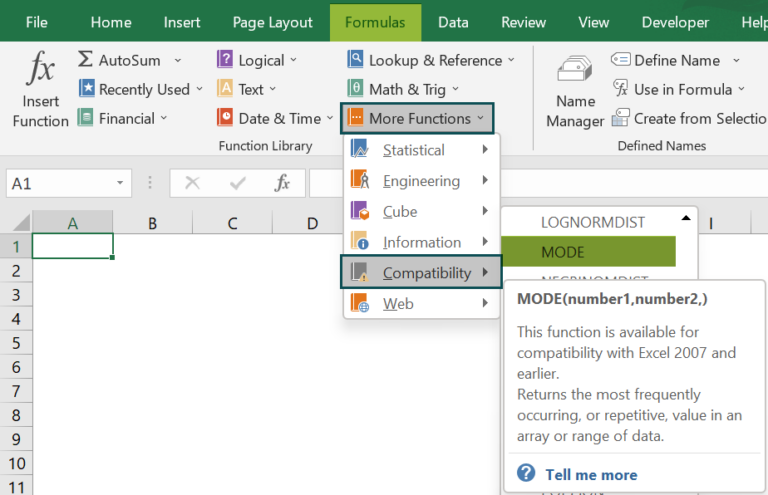And to use the latest version of the MODE(), MODE.SNGL() and MODE.MULT(), click FormulasMore FunctionsStatistical.

2. Why is the MODE function in Excel not working?

The MODE function in Excel may not work because of the below reasons:

The MODE() arguments are not numeric values.
The MODE() arguments contain error values or texts that do not translate into numeric values.
The source data set does not contain reoccurring data points.
All data points in the given data set are text, logical values, or empty cells.

3. How to use the MODE() in Excel VBA?

We can use the MODE() in Excel VBA using the below method:
Application.WorksheetFunction.Mode()

Let us see how to use it in Excel VBA with an example.

The table below shows a grocery items list and the corresponding count of units delivered in columns A and B.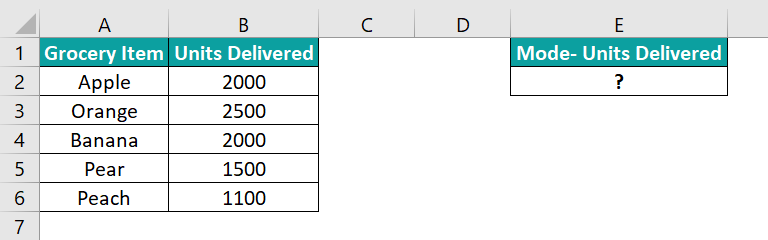Suppose we have to determine the mode of units delivered and display the result in cell E2. Then the steps are:

Step 1: With the active sheet containing the above table, press the keys Alt + F11 to open the VBA Editor.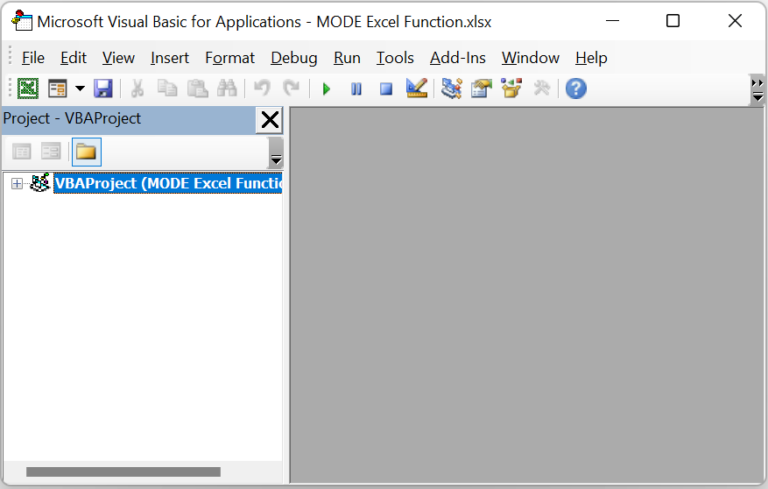Step 2: Choose the required VBAProject and select InsertModule to open the Module1 window.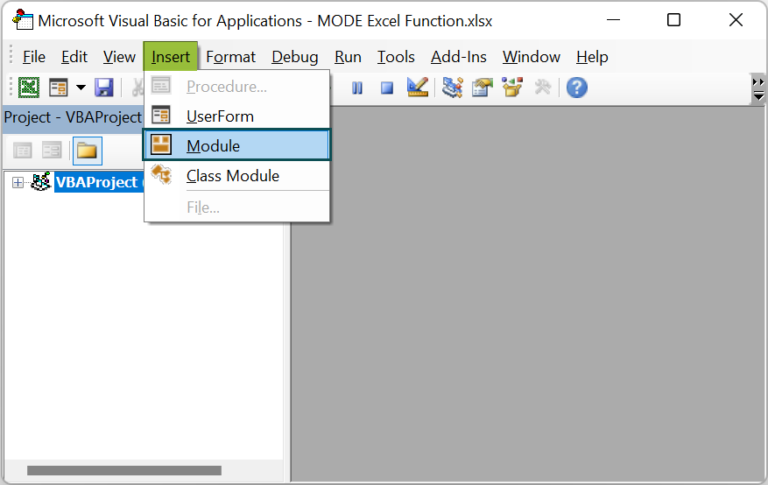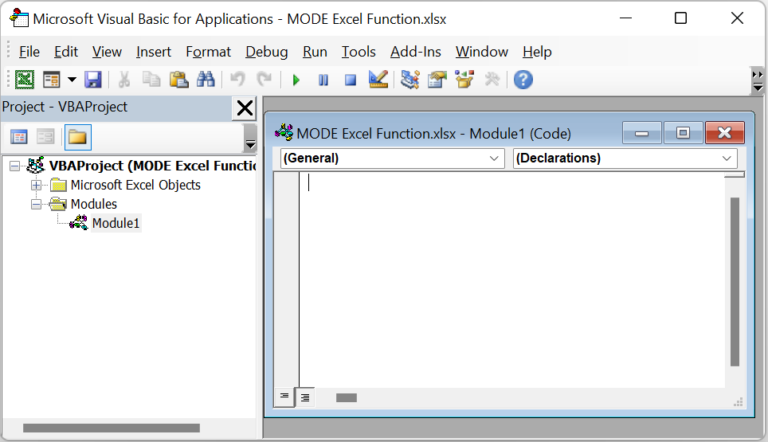Step 3: Enter the VBA code containing the MODE function in the Module1 window to determine the mode of units of grocery items delivered.

Sub mode_fn()
Range(“E2”) = Application.WorksheetFunction.Mode(Range(“B2:B6”))
End Sub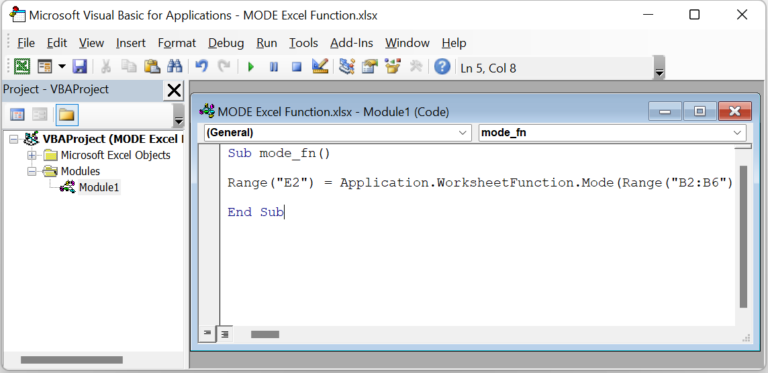Step 4: Now click on the Run Sub/UserForm icon in the top menu to execute the code.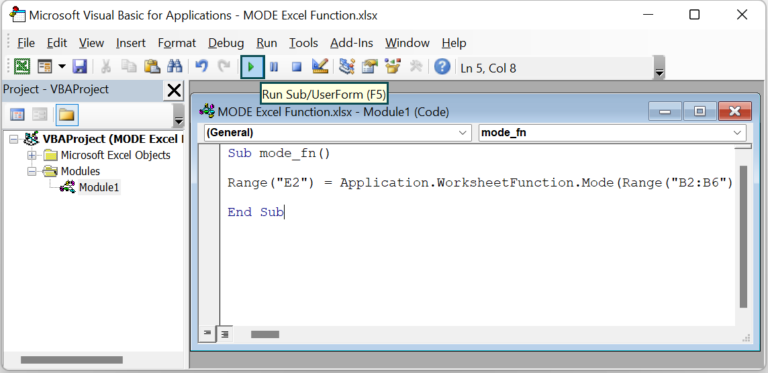And finally, if we open the active worksheet after the code gets executed, we will see the required mode value, 2000, in the target cell E2.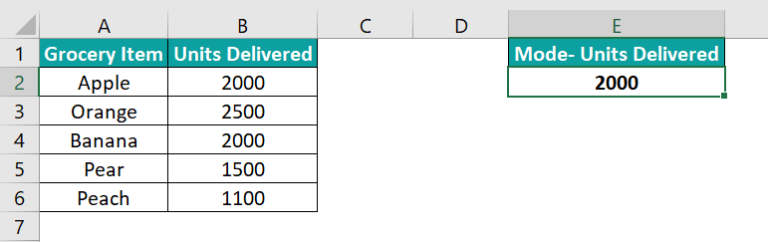In the above example, the MODE() in Excel VBA checks the supplied array range for the most frequently occurring values. And as the value 2000 appears twice in the given cell range, B2:B6, the VBA code output is 2000.